Question

Write program in C

Question 1 •

try to implement median3() function and trace what happened to array.

median3 (int array[], int left, int right){

// to do …

}

Question 2.

•Try to implement quicksort() partition phase.

quicksort(int array[], int left, int right){

// to do…
}

Question 3.

Try to finish the whole process of quicksort

1.

```import java.util.Random;
public class QsortTest
{
public static void main (String [] args)
{
Comparable [] a;
Random   ran;

System.out.println("How many randomly generated integers to sort?");
int n = console.readInt();

ran  = new Random();
a    = new Comparable[n]; // get array of this number of objects
for (int i=0;i<n;i++)
{
a[i] = new Integer(ran.nextInt(100));
}

System.out.println("\nOriginal Array:");
printArray(a);

quicksort(a);

System.out.println("\n\nSorted Array:");
printArray(a);
} // end main

// --------------------------------------------------------------

public static void printArray(Comparable [] a)
{
for (int i=0;i<a.length;i++){
int j = ((Integer) a[i]).intValue();
System.out.print(j + " ");
}
System.out.println();
}

// --------------------------------------------------------------
// driver to recursive sort array of Comaprables using quicksort
// with median of 3 partition. Use insertion sort for small arrays

public static void quicksort(Comparable [] a)
{
quicksort(a,0,a.length-1);
}

private static void quicksort(Comparable [] a, int left, int right)
{
final int CUTOFF = 3;
if (right-left+1 < CUTOFF) { // need at least 3 elements for qs
insertionSort(a,left,right);
}
else {
Comparable pivot = median3(a,left,right); // get pivot

int i = left, j = right-1;                // do the partitioning
while (i < j){
while (a[++i].compareTo(pivot) < 0) {}; // advance left ptr
while (a[--j].compareTo(pivot) > 0) {}; // decrement rt ptr
swap(a,i,j);
} // end while

swap(a,i,j);        // undo last (incorrect) swap
swap(a,i, right-1);   // restore pivot

quicksort(a,left,i-1);   // recursive calls on smalelr
quicksort(a,i+1,right);  // and larger subarrays
} // end else

} // end quicksort

// --------------------------------------------------------------

// median of 3 partitioning - put the 3 in order, and hide pivot
private static Comparable median3(Comparable [] a, int left, int right)
{
int center = (left+right)/2;
if (a[center].compareTo(a[left]) < 0)
swap(a,left,center);

if (a[right].compareTo(a[left]) < 0)
swap(a,left,right);

if (a[right].compareTo(a[center]) < 0)
swap(a,center,right);

// put pivot at NEXT to rightmost position
swap(a,center,right-1);
return a[right-1];    // return the pivot too

}

// --------------------------------------------------------------

// swap the elements at index i and j
private static void swap(Comparable a[], int i, int j)
{
Comparable tmp = a[i];
a[i] = a[j];
a[j] = tmp;
}

private static void insertionSort(Comparable a[], int left, int right)
{
int j;

for (int p=left+1; p<= right; p++){
Comparable tmp = a[p];
for (j=p; j>left && tmp.compareTo( a[j-1]) < 0; j--)
a[j] = a[j-1];
a[j] = tmp;
}

} // end insertionSort

} // end class QsortTest```

2.int partition(int[] A, int lower, int pivot_index, int upper)
//@requires 0 <= lower && lower <= pivot_index;
//@requires pivot_index < upper && upper <= \length(A);
//@ensures lower <= \result && \result < upper;
//@ensures ge_seg(A[\result], A, lower, \result);
//@ensures le_seg(A[\result], A, \result, upper);
{
// Hold the pivot element off to the left at "lower"
int pivot = A[pivot_index];
swap(A, lower, pivot_index);
int left = lower+1;
int right = upper;
while (left < right)
//@loop_invariant lower+1 <= left && left <= right && right <= upper;
//@loop_invariant ge_seg(pivot, A, lower+1, left); // Not lower!
//@loop_invariant le_seg(pivot, A, right, upper);
{
if (A[left] <= pivot) {
left++;
} else {
//@assert A[left] > pivot;
swap(A, left, right-1);
right--;
}
}
//@assert left == right;
swap(A, lower, left-1);
return left-1;
}

3.

void swap(int* a, int* b)
{
int t = *a;
*a = *b;
*b = t;
}

/* This function takes last element as pivot, places
the pivot element at its correct position in sorted
array, and places all smaller (smaller than pivot)
to left of pivot and all greater elements to right
of pivot */
int partition (int arr[], int low, int high)
{
int pivot = arr[high]; // pivot
int i = (low - 1); // Index of smaller element

for (int j = low; j <= high- 1; j++)
{
// If current element is smaller than or
// equal to pivot
if (arr[j] <= pivot)
{
i++; // increment index of smaller element
swap(&arr[i], &arr[j]);
}
}
swap(&arr[i + 1], &arr[high]);
return (i + 1);
}

/* The main function that implements QuickSort
arr[] --> Array to be sorted,
low --> Starting index,
high --> Ending index */
void quickSort(int arr[], int low, int high)
{
if (low < high)
{
/* pi is partitioning index, arr[p] is now
at right place */
int pi = partition(arr, low, high);

// Separately sort elements before
// partition and after partition
quickSort(arr, low, pi - 1);
quickSort(arr, pi + 1, high);
}
}

/* Function to print an array */
void printArray(int arr[], int size)
{
int i;
for (i=0; i < size; i++)
printf("%d ", arr[i]);
printf("n");
}

// Driver program to test above functions
int main()
{
int arr[] = {10, 7, 8, 9, 1, 5};
int n = sizeof(arr)/sizeof(arr);
quickSort(arr, 0, n-1);
printf("Sorted array: n");
printArray(arr, n);
return 0;
}
Output:

Sorted array:
1 5 7 8 9 10

#### Earn Coins

Coins can be redeemed for fabulous gifts.

Similar Homework Help Questions
• ### JAVA- Trace the recursive quick sort and partition methods in Lab6.java for this list of numbers:...

JAVA- Trace the recursive quick sort and partition methods in Lab6.java for this list of numbers: 47 71 15 35 66 61 44 26 68 56 18 19 36 84 69 55 1. Find the value of pivot 2. Show the result after partitionIt() is called first time 3. Show the value of partition 4. Show the content of the array ///////////////////////////// Lab6.java class ArrayIns { private long[] theArray; // ref to array theArray private int nElems; // number of...

• ### Trying to do this in C++ Question 1: 1. Implement the function: int minInArray (int arr[],...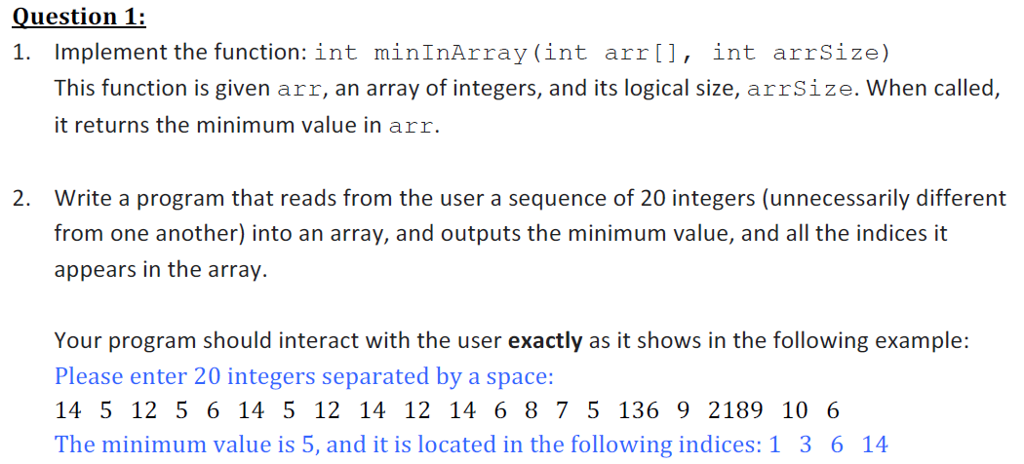Trying to do this in C++ Question 1: 1. Implement the function: int minInArray (int arr[], int arrSize) This function is given arr, an array of integers, and its logical size, arrSize. When called, t returns the minimum value in arr. Write a program that reads from the user a sequence of 20 integers (unnecessarily different from one another) into an array, and outputs the minimum value, and all the indices it appears in the array. 2. Your program should...

• ### c++ please read all question edit the program to test different random sizes of the array and give me the time in a file will be like random size of the array and next to it the time it took for each...

c++ please read all question edit the program to test different random sizes of the array and give me the time in a file will be like random size of the array and next to it the time it took for each size Im trying to do time analysis for Quick sort but i keep getting time = 0 also i want edit the program to test different random sizes of the array and give me the time in a...

• ### Done in C++ using visual studio 1. Write a program with one additional function called int[]...

Done in C++ using visual studio 1. Write a program with one additional function called int[] reverseArray(int array). This function will receive an array from main and then reverse and should return the reversed array to the main to print out. Use a single array and a single loop, you’re are neither allowed to print out just in reverse order nor allowed to use another array variable to store the original array in reverse order. 2. Write a program which...

• ### HW60.1. Array Quicksort You've done partition so now it's time to finish Quicksort. Create a publ...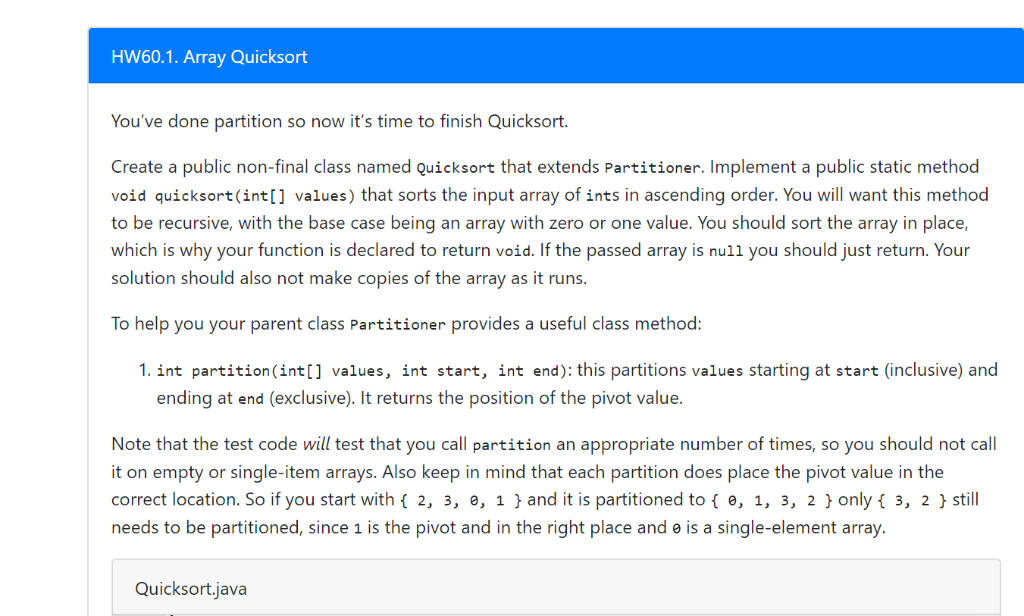HW60.1. Array Quicksort You've done partition so now it's time to finish Quicksort. Create a public non-final class named Quicksort that extends Partitioner. Implement a public static method void quicksort (int] values) that sorts the input array of ints in ascending order. You will want this method to be recursive, with the base case being an array with zero or one value. You should sort the array in place, which is why your function is declared to return void. If...

• ### this program is in C. Write a program that computes the number of elements in an...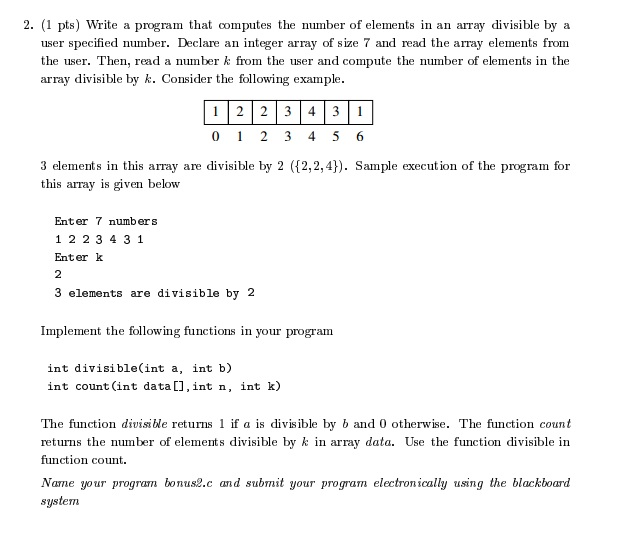this program is in C. Write a program that computes the number of elements in an array divisible by a user specified number. Declare an integer array of size 7 and read the array elements from the user. Then, read a number k from the user and compute the number of elements in the array divisible by k. Consider the following example. 3 elements in this array are divisible by 2 ({2,2,4}). Sample execution of the program for this array...

• ### I currently have this but it doesn't work for the three item arrays public class Quicksort...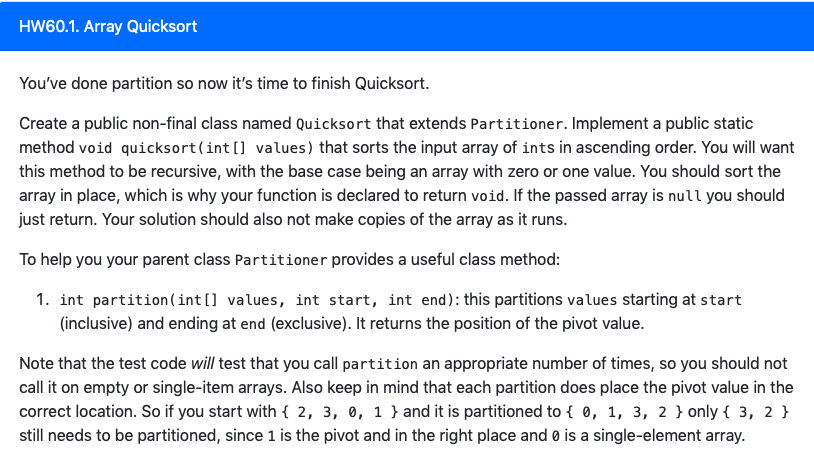I currently have this but it doesn't work for the three item arrays public class Quicksort extends Partitioner { public static void quicksort(int[] values) { if (values == null || values.length < 2) { return; } quicksort(values, 0, values.length - 1); } private static void quicksort(int[] values, int start, int end) { System.out.println(values); System.out.println(start); System.out.println(end); if (values == null || Math.abs(start - end) == 1) { return; } if (end > start) { int pivotValueIndex = partition(values, start, end); quicksort(values,...

• ### Write a c program to implement Quicksort for arrays of length 16, as described in the...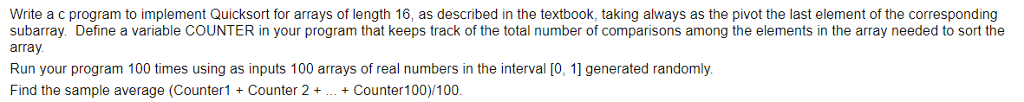Write a c program to implement Quicksort for arrays of length 16, as described in the textbook, taking always as the pivot the last element of the corresponding subarray. Define a variable COUNTER in your program that keeps track of the total number of comparisons among the elements in the array needed to sort the array Run your program 100 times using as inputs 100 arrays of real numbers in the interval [0, 11 generated randomly. Find the sample average...

• ### In C language Write a program that includes a function search() that finds the index of...

In C language Write a program that includes a function search() that finds the index of the first element of an input array that contains the value specified. n is the size of the array. If no element of the array contains the value, then the function should return -1. The program takes an int array, the number of elements in the array, and the value that it searches for. The main function takes input, calls the search()function, and displays...

• ### Suppose the quicksort program shown uses a partition function that always picks a[m] as the 5B....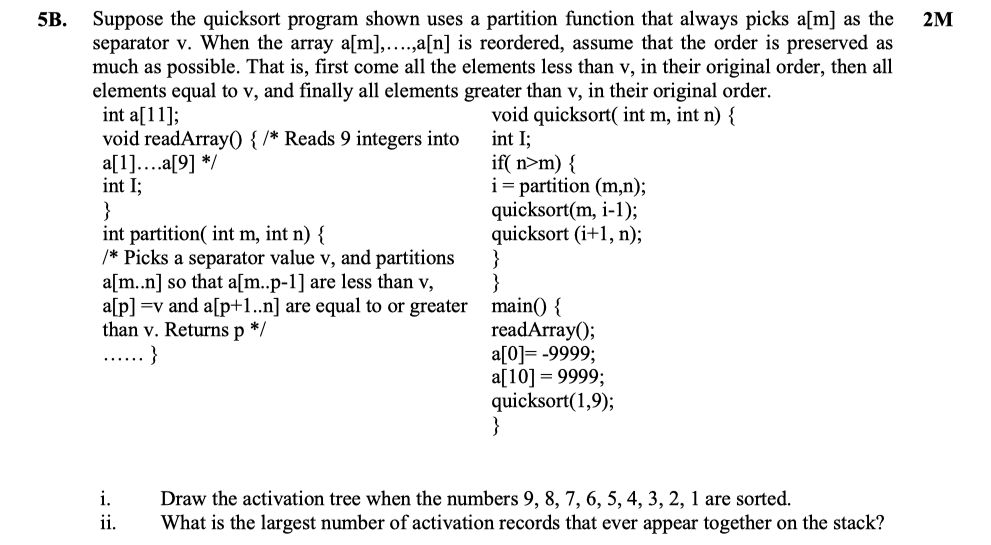Suppose the quicksort program shown uses a partition function that always picks a[m] as the 5B. 2M separator v. When the array a[m],....a[n] is reordered, assume that the order is preserved as much as possible. That is, first come all the elements less than v, in their original order, then all elements equal to v, and finally all elements greater than v, in their original order. int a; void readArray0 {/* Reads 9 integers into int I; void quicksort( int...